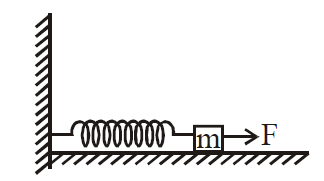# Q. A block of mass m, lying on a smooth horizontal surface, is attached to a spring (of negligible mass) of spring constant k. The other end of the spring is fixed, as shown in the figure. The block is initally at rest in its equilibrium position. If now the block is pulled with a constant force F, the maximum speed of the block is :JEE Main JEE Main 2019

Solution:

## Maximum speed is at mean position (equilibrium). F = kx $x = \frac{F}{k}$ $W_{F} + W_{sp} = \Delta KE$ $F\left(x\right) - \frac{1}{2} kx^{2} = \frac{1}{2} mv^{2} -0$ $F\left(\frac{F}{k}\right)- \frac{1}{2} k \left(\frac{F}{k}\right)^{2} = \frac{1}{2} mv^{2}$ $\Rightarrow v_{max } = \frac{F}{\sqrt{mk}}$

You must select option to get answer and solution

## 1. The potential to which a conductor is raised, depends on ........

KCET 2005 Electrostatic Potential And Capacitance

## 2. The refractive index of a particular material is 1.67 for blue light, 1.65 for yellow light and 1.63 for red light. The dispersive power of the material is .........

KCET 2004 Electrostatic Potential And Capacitance

## 3. In a permanent magnet at room temperature

KCET 2019 Moving Charges And Magnetism

## 4. An antenna uses electromagnetic waves of frequency 5 MHz. For proper working, the size of the antenna should be

KCET 2019 Communication Systems

## 5. In the following circuit, what are P and Q?

KCET 2019 Semiconductor Electronics: Materials Devices And Simple Circuits

## 6. Two metal plates are separated by 2 cm. The potentials of the plates are − 10 V and + 30 V. The electric field between the two plates is

KCET 2019 Electrostatic Potential And Capacitance

KCET 2019 Nuclei

## 8. For a transistor amplifier, the voltage gain

KCET 2019 Semiconductor Electronics: Materials Devices And Simple Circuits

## 9. “Parsec” is the unit of

BHU 2007 Physical World, Units and Measurements

## 10. “Parsec” is the unit of

BHU 2007 Physical World, Units and Measurements